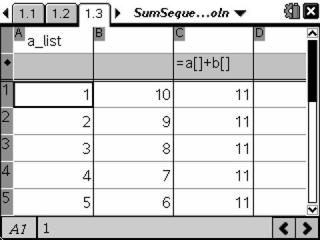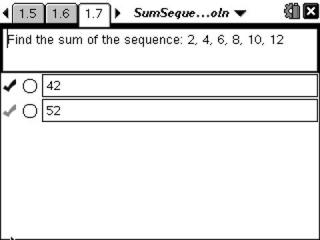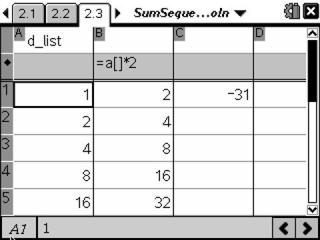# Activities

••• ##### Subject Area

• Math: Algebra II: Sequences

• ##### Author9-12

60 Minutes

• ##### Device
• TI-Nspire™ Navigator™
• TI-Nspire™
• TI-Nspire™ CAS
• ##### Software

TI-Nspire™
TI-Nspire™ CAS

2.0

## Sums of Sequences#### Activity Overview

In this activity, students will develop formulas for the sum of arithmetic and geometric sequences. Students will then find the sum of sequences using the formulas developed.

#### Key Steps

•In this activity, students will first perform a numerical process/proof to find the sum of an arithmetic sequence. To do this, they will be entering the sequence in reverse order and finding the sums of the rows.

•After students develop a formula for the sum of an arithmetic sequence, they will apply the formula.

•In the second part of the activity, students develop the formula for the sum of a geometric sequence. To do this, they will multiply each term in the sequence by the common ratio, find the difference between the two different values, and divide by a value to determine the sum of column A.